Скачать презентацию Public Key Cryptosystems RSA Diffie-Hellman Department of Computer

95ab6662062fc9b9177ab8d5d0ce85a1.ppt

• Количество слайдов: 14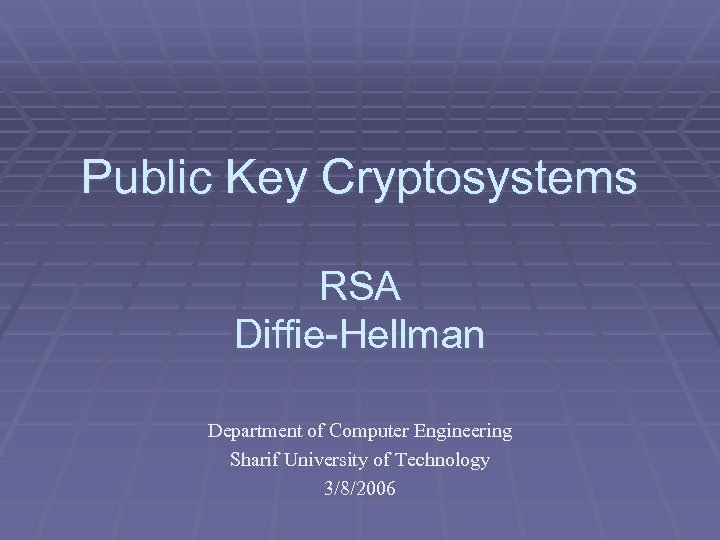Public Key Cryptosystems RSA Diffie-Hellman Department of Computer Engineering Sharif University of Technology 3/8/2006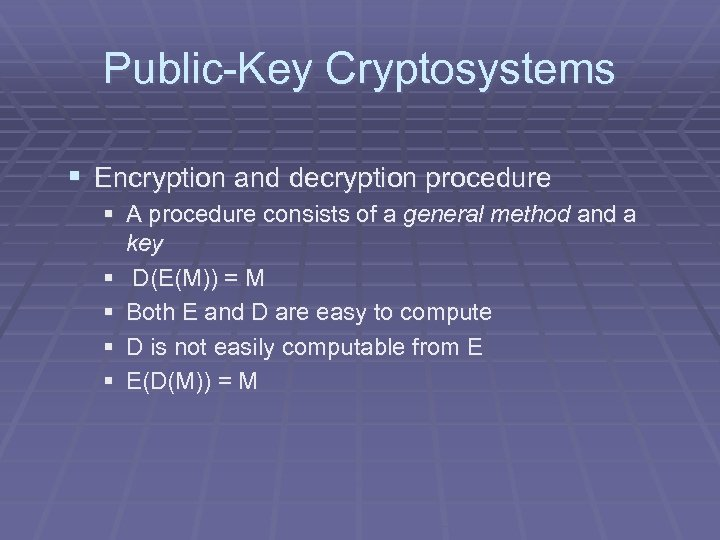Public-Key Cryptosystems § Encryption and decryption procedure § A procedure consists of a general method and a key § D(E(M)) = M § Both E and D are easy to compute § D is not easily computable from E § E(D(M)) = M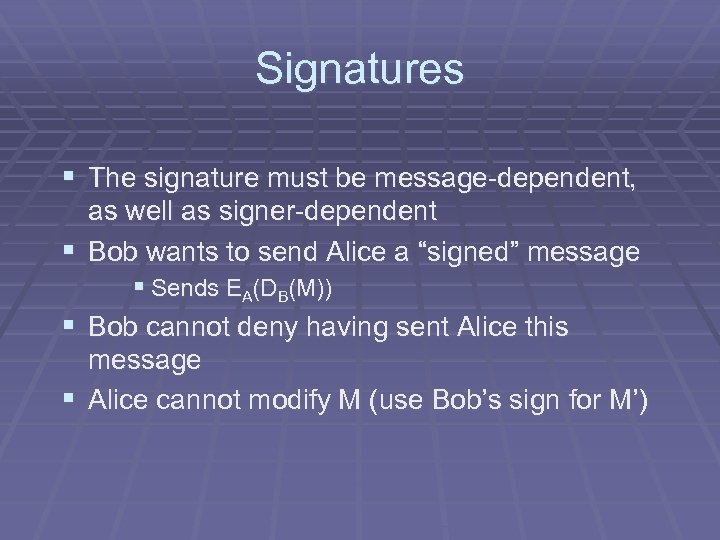Signatures § The signature must be message-dependent, § § § as well as signer-dependent Bob wants to send Alice a “signed” message § Sends EA(DB(M)) Bob cannot deny having sent Alice this message Alice cannot modify M (use Bob’s sign for M’)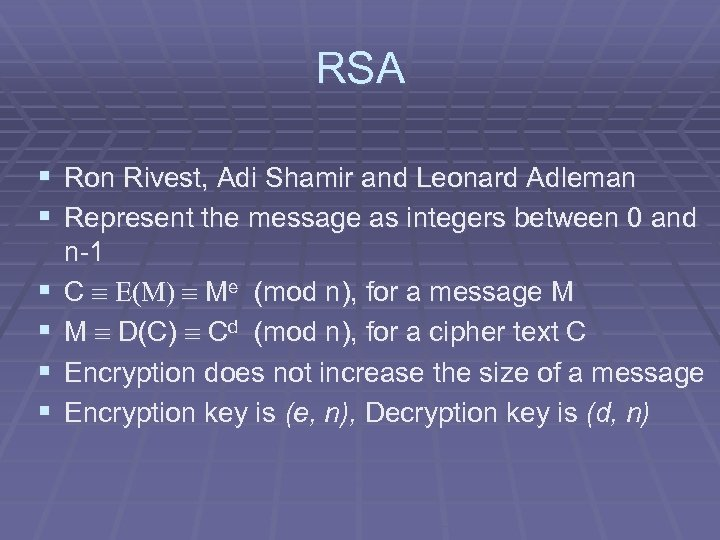RSA § Ron Rivest, Adi Shamir and Leonard Adleman § Represent the message as integers between 0 and § § n-1 C º E(M) º Me (mod n), for a message M M º D(C) º Cd (mod n), for a cipher text C Encryption does not increase the size of a message Encryption key is (e, n), Decryption key is (d, n)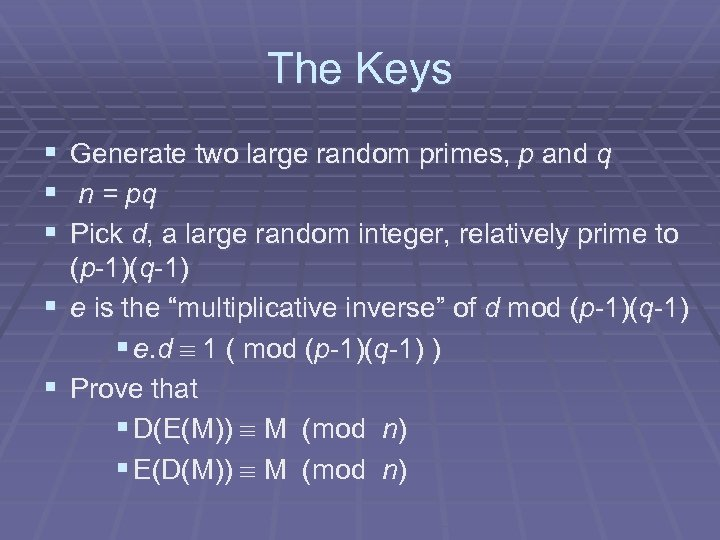The Keys § § § Generate two large random primes, p and q n = pq Pick d, a large random integer, relatively prime to (p-1)(q-1) § e is the “multiplicative inverse” of d mod (p-1)(q-1) § e. d º 1 ( mod (p-1)(q-1) ) § Prove that § D(E(M)) º M (mod n) § E(D(M)) º M (mod n)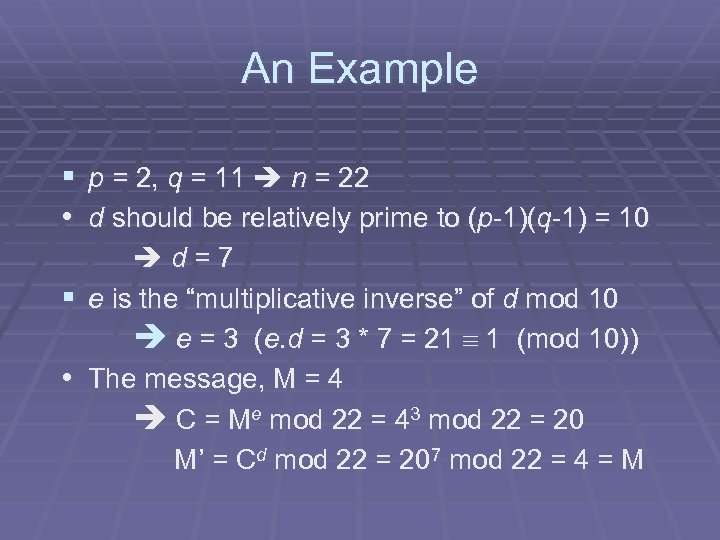An Example § p = 2, q = 11 n = 22 • d should be relatively prime to (p-1)(q-1) = 10 d=7 § e is the “multiplicative inverse” of d mod 10 e = 3 (e. d = 3 * 7 = 21 º 1 (mod 10)) • The message, M = 4 C = Me mod 22 = 43 mod 22 = 20 M’ = Cd mod 22 = 207 mod 22 = 4 = M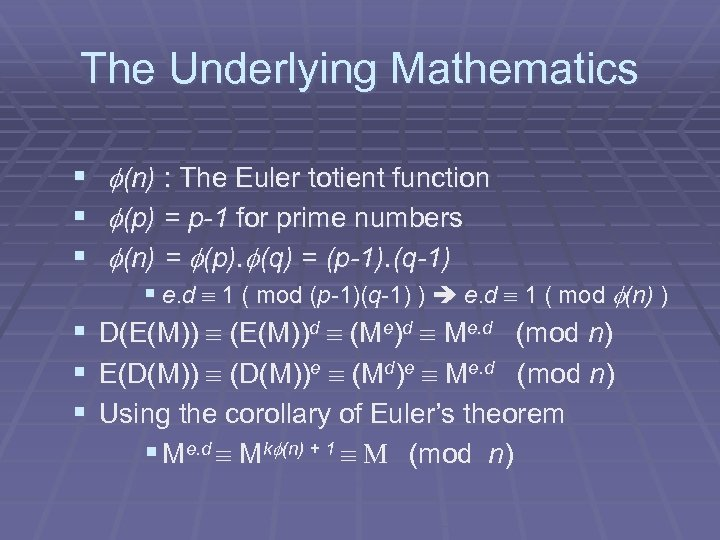The Underlying Mathematics § § § f(n) : The Euler totient function f(p) = p-1 for prime numbers f(n) = f(p). f(q) = (p-1). (q-1) § § e. d º 1 ( mod (p-1)(q-1) ) e. d º 1 ( mod f(n) ) D(E(M)) º (E(M))d º (Me)d º Me. d (mod n) E(D(M)) º (D(M))e º (Md)e º Me. d (mod n) Using the corollary of Euler’s theorem § Me. d º Mkf(n) + 1 º M (mod n)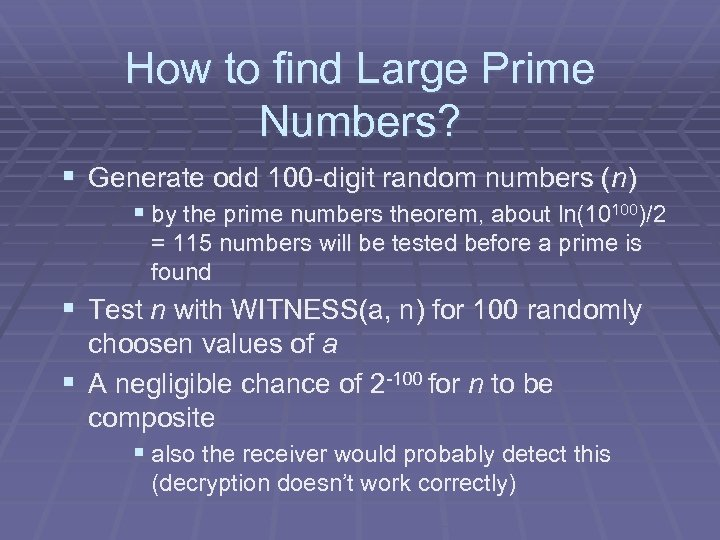How to find Large Prime Numbers? § Generate odd 100 -digit random numbers (n) § by the prime numbers theorem, about ln(10100)/2 = 115 numbers will be tested before a prime is found § Test n with WITNESS(a, n) for 100 randomly choosen values of a § A negligible chance of 2 -100 for n to be composite § also the receiver would probably detect this (decryption doesn’t work correctly)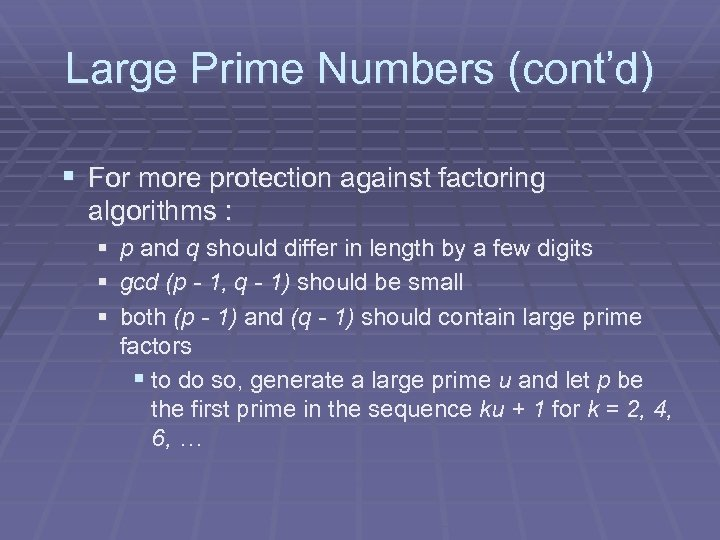Large Prime Numbers (cont’d) § For more protection against factoring algorithms : § § § p and q should differ in length by a few digits gcd (p - 1, q - 1) should be small both (p - 1) and (q - 1) should contain large prime factors § to do so, generate a large prime u and let p be the first prime in the sequence ku + 1 for k = 2, 4, 6, …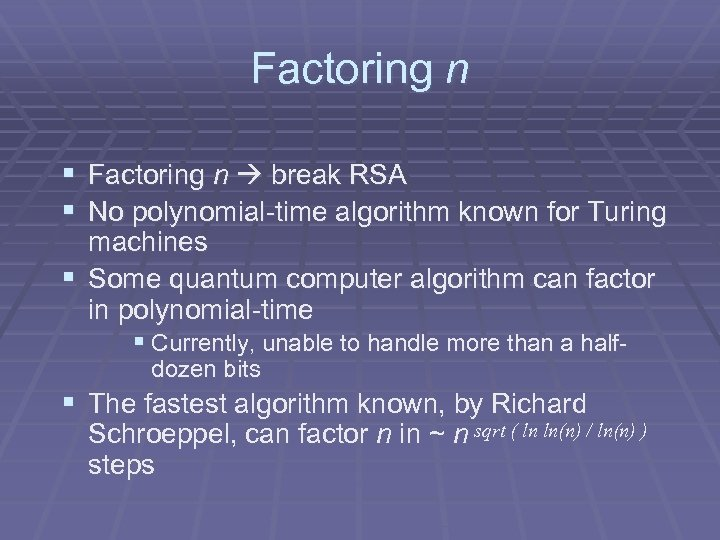Factoring n § Factoring n break RSA § No polynomial-time algorithm known for Turing machines § Some quantum computer algorithm can factor in polynomial-time § Currently, unable to handle more than a halfdozen bits § The fastest algorithm known, by Richard Schroeppel, can factor n in ~ n sqrt ( ln ln(n) / ln(n) ) steps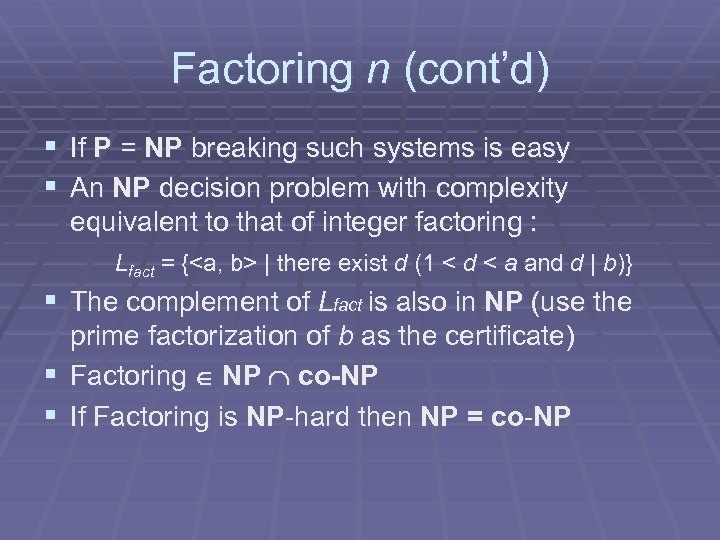Factoring n (cont’d) § If P = NP breaking such systems is easy § An NP decision problem with complexity equivalent to that of integer factoring : Lfact = { | there exist d (1 < d < a and d | b)} § The complement of Lfact is also in NP (use the prime factorization of b as the certificate) § Factoring NP co-NP § If Factoring is NP-hard then NP = co-NP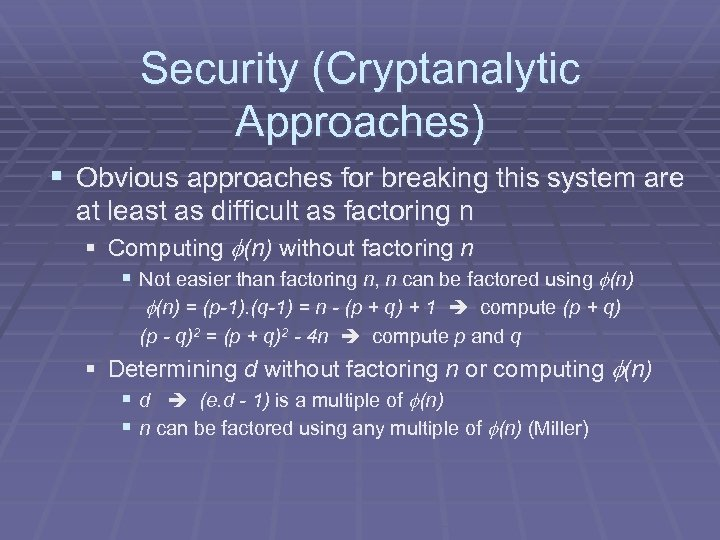Security (Cryptanalytic Approaches) § Obvious approaches for breaking this system are at least as difficult as factoring n § Computing f(n) without factoring n § Not easier than factoring n, n can be factored using f(n) = (p-1). (q-1) = n - (p + q) + 1 compute (p + q) (p - q)2 = (p + q)2 - 4 n compute p and q § Determining d without factoring n or computing f(n) § d (e. d - 1) is a multiple of f(n) § n can be factored using any multiple of f(n) (Miller)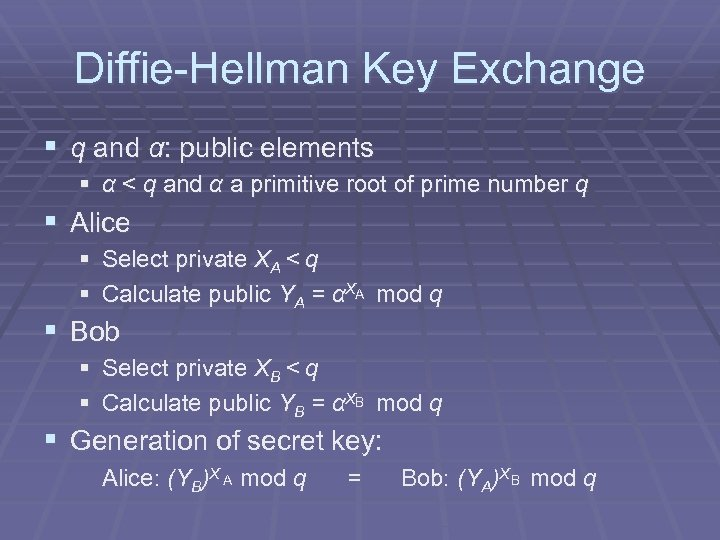Diffie-Hellman Key Exchange § q and α: public elements § α < q and α a primitive root of prime number q § Alice § Select private XA < q § Calculate public YA = αXA mod q § Bob § Select private XB < q § Calculate public YB = αXB mod q § Generation of secret key: Alice: (YB)X A mod q = Bob: (YA)X B mod q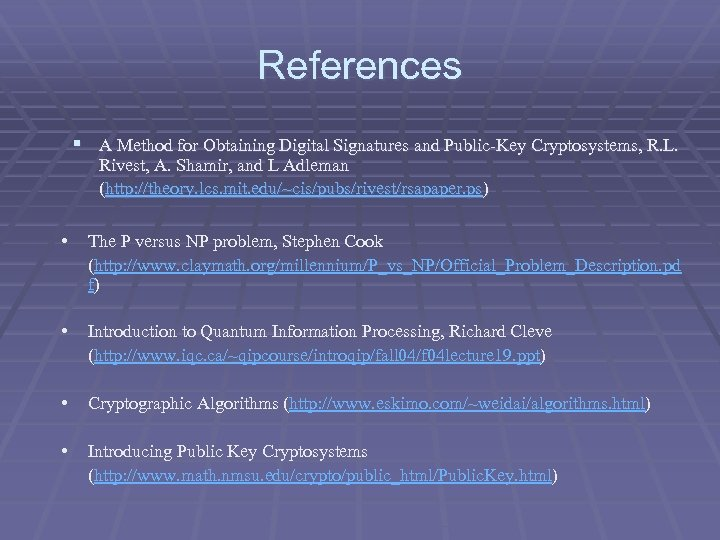References § A Method for Obtaining Digital Signatures and Public-Key Cryptosystems, R. L. Rivest, A. Shamir, and L Adleman (http: //theory. lcs. mit. edu/~cis/pubs/rivest/rsapaper. ps) • The P versus NP problem, Stephen Cook (http: //www. claymath. org/millennium/P_vs_NP/Official_Problem_Description. pd f) • Introduction to Quantum Information Processing, Richard Cleve (http: //www. iqc. ca/~qipcourse/introqip/fall 04/f 04 lecture 19. ppt) • Cryptographic Algorithms (http: //www. eskimo. com/~weidai/algorithms. html) • Introducing Public Key Cryptosystems (http: //www. math. nmsu. edu/crypto/public_html/Public. Key. html)